# Practice Quiz in Field Effect Transistor Amplifiers Part 1

(Last Updated On: February 3, 2020)

This is the Online Practice Quiz in Field Effect Transistor Amplifiers Part 1 from the book, Electronic Devices and Circuit Theory 10th Edition by Robert L. Boylestad. If you are looking for a reviewer in Electronics Engineering this will definitely help. I can assure you that this will be a great help in reviewing the book in preparation for your Board Exam. Make sure to familiarize each and every questions to increase the chance of passing the ECE Board Exam.

### Start Part I of the Online Practice Quiz

#### Quiz in Field Effect Transistor Amplifiers

Question 1. Referring to this figure, calculate Av if yos = 20 µS.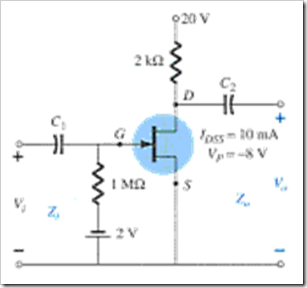A. –3.48

B. –3.56

C. –3.62

D. –4.02

Question 2. Referring to this figure, calculate Zo if yos = 40 µS.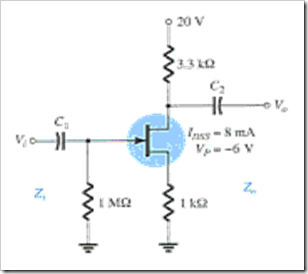A. 2.92 kΩ

B. 3.20 kΩ

C. 3.25 kΩ

D. 3.75 kΩ

Question 3. Referring to this figure, obtain gm for ID = 6 mA.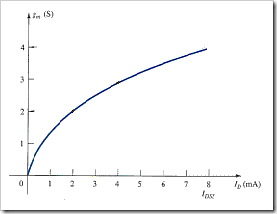A. 2.83 mS

B. 3.00 mS

C. 3.25 mS

D. 3.46 mS

Question 4. Where do you get the level of gm and rd for an FET transistor?

A. from the dc biasing arrangement

B. from the specification sheet

C. from the characteristics

D. All of these

Question 5. Calculate gm and rd if yfs = 4 mS and yos = 15 ΩS.

A. 4 mS, 66.7 kΩ

B. 4 mS, 15 kΩ

C. 66.7 kΩ, 4 mS

D. None of these

Question 6. What is the range of gm for JFETs?

A. 1 µS to 10 µS

B. 100 µS to 1000 µS

C. 1000 µS to 5000 µS

D. 10000 µS to 100000 µS

Question 7. What is (are) the function(s) of the coupling capacitors C1 and C2 in an FET circuit?

A. to create an open circuit for dc analysis

B. to isolate the dc biasing arrangement from the applied signal and load

C. to create a short-circuit equivalent for ac analysis

D. All of these

Question 8. Referring to this figure, calculate Zo for VGSQ = –3.2 V.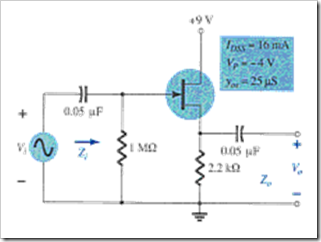A. 362.52 Ω

B. 340.5 Ω

C. 420.5 Ω

D. 480.9 Ω

Question 9. Referring to the following figure, calculate gm for VGSQ = –1.25 V.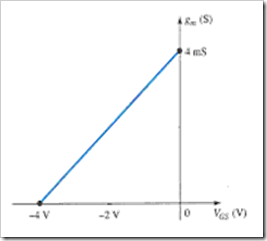A. 2 mS

B. 2.5 mS

C. 2.75 mS

D. 3.25 mS

Question 10. FET amplifiers provide ________.

A. excellent voltage gain

B. high input impedance

C. low power consumption

D. All of these

### More Practice Quiz in Field Effect Transistor Amplifiers

Practice Quiz Part 1

Practice Quiz Part 2

Practice Quiz Part 3

Practice Quiz Part 4

Practice Quiz Part 5

Practice Quiz Part 6

### See: Complete List of Practice Quizzes

Note: After taking this particular quiz, you can proceed to check all the topics.